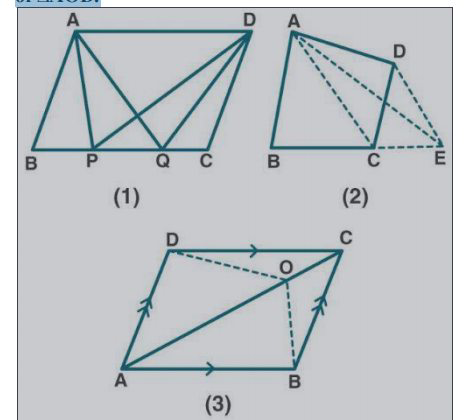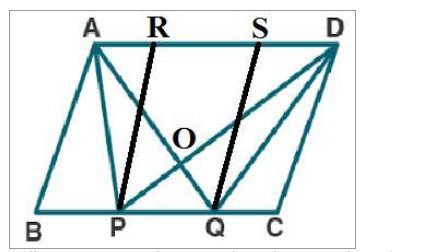# ML Aggarwal Solutions Class 9 Mathematics Solutions for Theorems on Area Exercise 14 in Chapter 14 - Theorems on Area

(a) In the figure (1) given below, ABCD is a parallelogram. Points P and Q on

BC trisect BC into three equal parts. Prove that :

area of ∆APQ = area of ∆DPQ = 1/6 (area of ||gm ABCD)

(b) In the figure (2) given below, DE is drawn parallel to the diagonal AC of the

quadrilateral ABCD to meet BC produced at the point E. Prove that area of quad.

ABCD = area of ∆ABE.

(c) In figure (3) given below, ABCD is a parallelogram. O is any point on the

diagonal AC of the parallelogram. Show that the area of ∆AOB is equal to the area

of ∆AOD.(a) Given:

From fig (1)In ||gm ABCD, points P and Q trisect BC into three equal parts.

To prove:

area of ∆APQ = area of ∆DPQ = 1/6 (area of ||gm ABCD)

Firstly, let us construct: through P and Q, draw PR and QS parallel to AB and CD.

Proof:

ar (∆APD) = ar (∆AQD) [Since ∆APD and ∆AQD lie on the same base AD and between

the same parallel lines AD and BC]

ar (∆APD) – ar (∆AOD) = ar (∆AQD) – ar (∆AOD) [On subtracting ar ∆AOD on both

sides]

ar (∆APO) = ar (∆OQD) ….. (1)

ar (∆APO) + ar (∆OPQ) = ar (∆OQD) + ar (∆OPQ) [On adding ar ∆OPQ on both sides]

ar (∆APQ) = ar (∆DPQ) …… (2)

We know that ∆APQ and ||gm PQSR are on the same base PQ and between the same parallel

ar (∆APQ) = ½ ar (||gm PQRS) …… (3)

Now,

[ar (||gm ABCD)/ar (||gm PQRS)] = [(BC×height)/(PQ×height)] =

[(3PQ×height)/(1PQ×hight)]

ar (||gm PQRS) = 1/3 ar (||gm ABCD) …. (4)

by using (2), (3), (4), we get

ar (∆APQ) = ar (∆DPQ)

= ½ ar (||gm PQRS)

= ½ × 1/3 ar (||gm ABCD)

= 1/6 ar (||gm ABCD)

Hence proved.

(b) Given:

In the figure (2) given below, DE || AC the diagonal of the quadrilateral ABCD to meet at

point E on producing BC. Join AC, AE.ML Aggarwal Solutions for Class 9 Maths

Chapter 14 – Theorems on Area

To prove:

area of quad. ABCD = area of ∆ABE

Proof:

We know that ∆ACE and ∆ADE are on the same base AC and between the same

parallelogram.

Now by adding ar (∆ABC) on both sides, we get

ar (∆ACE) + ar (∆ABC) = ar (∆ADC) + ar (∆ABC)

ar (∆ ABE) = ar quad. ABCD

Hence proved.

(c) Given:

From fig (3)

In ||gm ABCD, O is any point on diagonal AC.

To prove:

area of ∆AOB is equal to the area of ∆AOD

Proof:

Let us join BD which meets AC at P.

In ∆ABD, AP is the median.

ar (∆ABP) = ar (∆ADP) ….. (1)

similarly, ar (∆PBO) = ar (∆PDO) …. (2)

now add (1) and (2), we get

ar (∆ABO) = ar (∆ADO) …. (3)

so,

∆AOB = ar ∆AOD

Hence proved.

Related Questions
Exercises

Lido

Courses

Teachers

Book a Demo with us

Syllabus

Maths
CBSE
Maths
ICSE
Science
CBSE

Science
ICSE
English
CBSE
English
ICSE
Coding

Terms & Policies

Selina Question Bank

Maths
Physics
Biology

Allied Question Bank

Chemistry
Connect with us on social media!